Home Practice
For learners and parents For teachers and schools
Textbooks
Full catalogue
Pricing SupportLog in

We think you are located in United States. Is this correct?

## Worked example 6: Inverse of the function $$y=a{x}^{2}$$

Determine the inverse of the quadratic function $$h(x) = 3x^{2}$$ and sketch both graphs on the same system of axes.

### Determine the inverse of the given function $$h(x)$$

• Interchange $$x$$ and $$y$$ in the equation.
• Make $$y$$ the subject of the new equation.
\begin{align*} \text{Let } y & = 3x^{2} \\ \text{Interchange } x \text{ and } y: \quad x & = 3y^{2} \\ \frac{x}{3} & = y^{2} \\ \therefore y & = ±\sqrt{\frac{x}{3}} \qquad (x \geq 0) \end{align*}

### Sketch the graphs on the same system of axes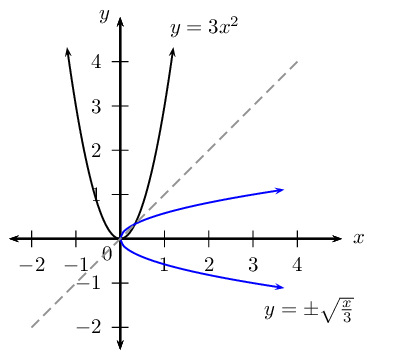Notice that the inverse does not pass the vertical line test and therefore is not a function.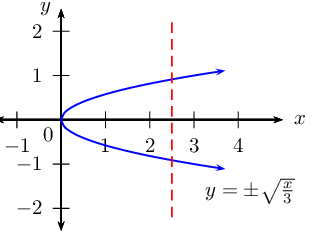To determine the inverse function of $$y=ax^{2}$$:

$\begin{array}{rll} &(1) \quad \text{Interchange } x \text{ and } y: & x = ay^{2} \\ &(2) \quad \text{Make } y \text{ the subject of the equation}: & \frac{x}{a} = y^{2} \\ &&\therefore y = ±\sqrt{\frac{x}{a}} \qquad (x \geq 0) \end{array}$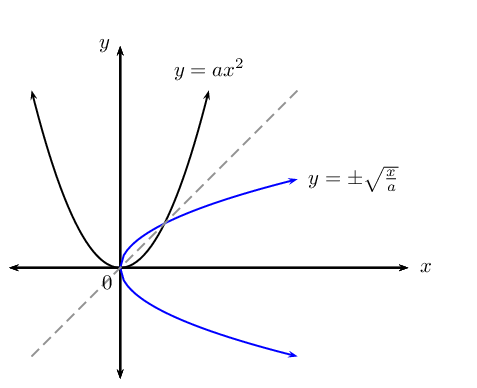The vertical line test shows that the inverse of a parabola is not a function. However, we can limit the domain of the parabola so that the inverse of the parabola is a function.

Domain and range

Consider the previous worked example $$h(x) = 3x^{2}$$ and its inverse $$y = ±\sqrt{\frac{x}{3}}$$:

• If we restrict the domain of $$h$$ so that $$x\ge 0$$, then $$h^{-1}(x) = \sqrt{\frac{x}{3}}$$ passes the vertical line test and is a function.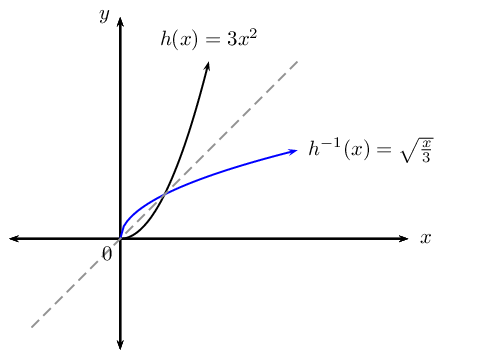• If the restriction on the domain of $$h$$ is $$x\le 0$$, then $$h^{-1}(x) = -\sqrt{\frac{x}{3}}$$ would also be a function.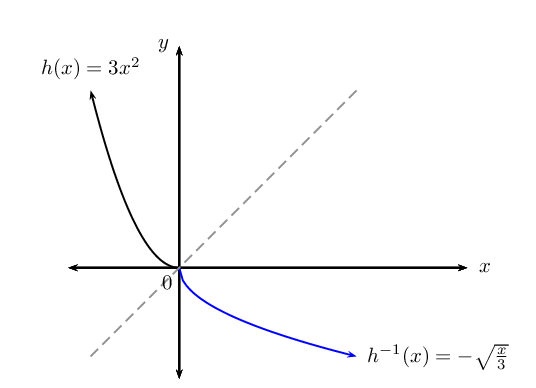The domain of the function is equal to the range of the inverse. The range of the function is equal to the domain of the inverse.

Similarly, a restriction on the domain of the function results in a restriction on the range of the inverse and vice versa.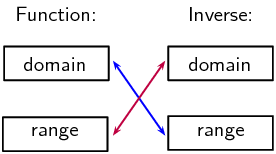## Worked example 7: Inverses - domain, range and restrictions

Determine the inverse of $$q(x) = 7x^{2}$$ and sketch both graphs on the same system of axes. Restrict the domain of $$q$$ so that the inverse is a function.

### Examine the function and determine the inverse

Determine the inverse of the function:

\begin{align*} \text{Let } y & = 7x^{2} \\ \text{Interchange } x \text{ and } y: \quad x & = 7y^{2} \\ \frac{x}{7} & = y^{2} \\ \therefore y & = ±\sqrt{\frac{x}{7}} \qquad (x \geq 0) \end{align*}

### Sketch both graphs on the same system of axes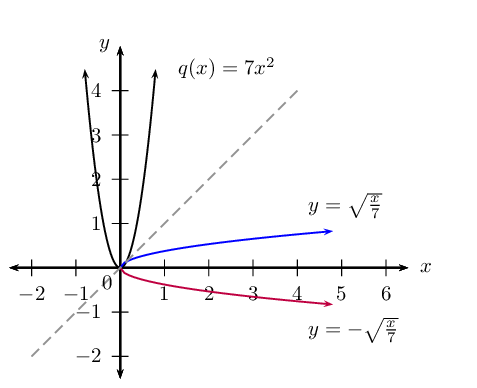### Determine the restriction on the domain

Option $$\text{1}$$: Restrict the domain of $$q$$ to $$x \ge 0$$ so that the inverse will also be a function $$\left( q^{-1} \right)$$. The restriction $$x \ge 0$$ on the domain of $$q$$ will restrict the range of $$q^{-1}$$ such that $$y \ge 0$$.

\begin{align*} q: \qquad & \text{domain } x \ge 0 \quad \text{range } y \ge 0 \\ q^{-1}: \qquad & \text{domain } x \ge 0 \quad \text{range } y \ge 0 \end{align*}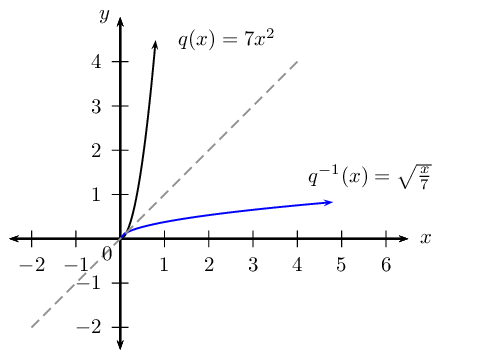or

Option $$\text{2}$$: Restrict the domain of $$q$$ to $$x \le 0$$ so that the inverse will also be a function $$\left( q^{-1} \right)$$. The restriction $$x \le 0$$ on the domain of $$q$$ will restrict the range of $$q^{-1}$$ such that $$y \le 0$$.

\begin{align*} q: \qquad & \text{domain } x \le 0 \quad \text{range } y \ge 0 \\ q^{-1}: \qquad & \text{domain } x \ge 0 \quad \text{range } y \le 0 \end{align*}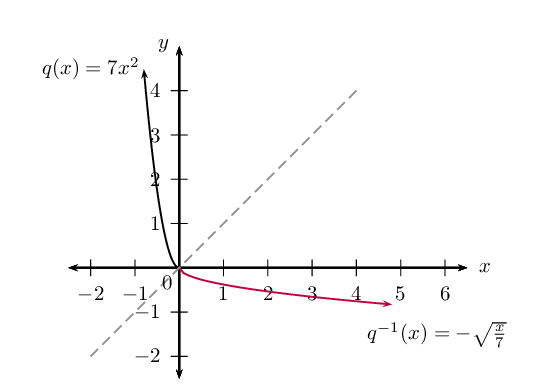## Worked example 8: Inverses - domain, range and restrictions

1. Determine the inverse of $$f(x) = -x^{2}$$.
2. Sketch both graphs on the same system of axes.
3. Restrict the domain of $$f$$ so that its inverse is a function.

### Determine the inverse of the function

\begin{align*} \text{Let } y & = -x^{2} \\ \text{Interchange } x \text{ and } y: \quad x & = -y^{2} \\ -x & = y^{2} \\ y & = ±\sqrt{-x} \qquad (x \leq 0) \end{align*}

Note: $$\sqrt{-x}$$ is only defined if $$x \le 0$$.

### Sketch both graphs on the same system of axes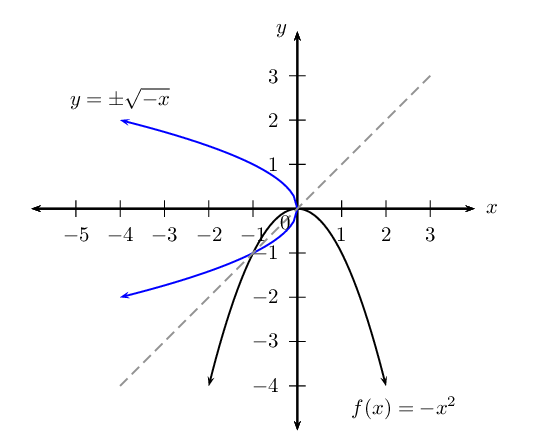The inverse does not pass the vertical line test and is not a function.

### Determine the restriction on the domain

• If $$f(x) = -x^{2}, \text{ for } x \le 0$$: \begin{align*} f: \qquad & \text{domain } x \le 0 \quad \text{range } y \le 0 \\ f^{-1}: \qquad & \text{domain } x \le 0 \quad \text{range } y \le 0 \end{align*}
• If $$f(x) = -x^{2}, \text{ for } x \ge 0$$: \begin{align*} f: \qquad & \text{domain } x \ge 0 \quad \text{range } y \le 0 \\ f^{-1}: \qquad & \text{domain } x \le 0 \quad \text{range } y \ge 0 \end{align*}

## Inverses - domain, range, intercepts, restrictions

Textbook Exercise 2.4

Determine the inverse for each of the following functions:

$$y = \frac{3}{4}x^{2}$$
\begin{align*} y & = \frac{3}{4}x^{2} \\ \text{Interchange } x \text{ and } y: \quad x & = \frac{3}{4}y^{2} \\ \frac{4}{3}x & = y^{2} \\ \therefore y & = \pm \sqrt{\frac{4}{3}x} \qquad (x \geq 0) \end{align*}
$$4y - 8x^{2} = 0$$
\begin{align*} \text{Interchange } x \text{ and } y: \quad 4x - 8y^{2} & = 0 \\ 4x & = 8y^{2} \\ \frac{1}{2}x & = y^{2} \\ \therefore y & = \pm \sqrt{\frac{1}{2}x} \qquad (x \geq 0) \end{align*}
$$x^{2} + 5y = 0$$
\begin{align*} \text{Interchange } x \text{ and } y: \quad y^{2} + 5x & = 0 \\ y^{2} & = - 5x \\ \therefore y & = \pm \sqrt{- 5x} \qquad (x \leq 0) \end{align*}
$$4y - 9 = (x + 3)(x - 3)$$
\begin{align*} 4y - 9 &= x^{2} - 9 \\ 4y &= x^{2} \\ \text{Interchange } x \text{ and } y: \quad 4x & = y^{2} \\ \therefore y & = \pm \sqrt{4x} \qquad (x \geq 0) \end{align*}

Given the function $$g(x) = \frac{1}{2}x^{2}$$ for $$x \ge 0$$.

Find the inverse of $$g$$.

\begin{align*} \text{Let } y & = \frac{1}{2}x^{2} \qquad (x \geq 0) \\ \text{Interchange } x \text{ and } y: \quad x & = \frac{1}{2}y^{2} \qquad (y \geq 0) \\ 2x & = y^{2} \\ y & = \sqrt{2x} \qquad (x \geq 0,y \geq 0) \\ & \\ \therefore g^{-1}(x) & = \sqrt{2x} \qquad (x \geq 0) \end{align*}

Draw $$g$$ and $$g^{-1}$$ on the same set of axes.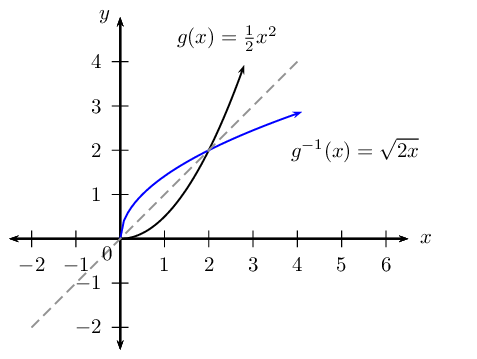Is $$g^{-1}$$ a function? Explain your answer.

Yes. It passes the vertical line test and is a one-to-one relation.

State the domain and range for $$g$$ and $$g^{-1}$$.

\begin{align*} g: \qquad & \text{domain } x \ge 0 \quad \text{range } y \ge 0 \\ g^{-1}: \qquad & \text{domain } x \ge 0 \quad \text{range } y \ge 0 \end{align*}

Determine the coordinates of the point(s) of intersection of the function and its inverse.

To find the points of intersection, we equate $$g(x)$$ and $$g^{-1}(x)$$:

\begin{align*} \frac{1}{2}x^{2} & = \sqrt{2x} \\ \left( \frac{1}{2}x^{2} \right)^{2} & = \left( \sqrt{2x} \right)^{2} \\ \frac{1}{4}x^{4} & = 2x \\ x^{4} & = 8x \\ x^{4} - 8x & = 0\\ x(x^{3} - 8) & = 0\\ x(x-2)(x^{2} + 2x + 4) & = 0\\ \therefore x = 0 \text{ or } x =2 \text{ or } & x^{2} + 2x + 4 = 0 \end{align*} \begin{align*} \text{If } x & = 0 \\ y & = 0 \\ \text{If } x & = 2 \\ y & = \frac{1}{2}(2)^{2} \\ & = 2 \end{align*} \begin{align*} \text{If } x^{2} + 2x + 4 & = 0 \\ \text{Use the quadratic formula } x & = \frac{-b \pm \sqrt{b^{2} - 4ac}}{2a} \\ & = \frac{-2 \pm \sqrt{(2)^{2} - 4(1)(4)}}{2(1)} \\ & = \frac{-2 \pm \sqrt{-12}}{2} \\ & = \text{no real solution} \end{align*}

Therefore, we get the points $$(0;0)$$ or $$(2;2)$$.

Given the graph of the parabola $$f(x) = ax^{2}$$ with $$x \ge 0$$ and passing through the point $$P(1;-3)$$.Determine the equation of the parabola.

\begin{align*} \text{Let } y & = ax^{2} \\ \text{Substitute } P(1;-3): \quad & -3 = a(1)^{2} \\ \therefore a & = -3 \\ \therefore f(x) & = -3x^{2} \quad (x \ge 0) \end{align*}

State the domain and range of $$f$$.

$$f: \enspace \text{domain } \{x: x \ge 0 \} \quad \text{range } \{y: y \le 0 \}$$

Give the coordinates of the point on $$f^{-1}$$ that is symmetrical to the point $$P$$ about the line $$y=x$$.

$$(-3;1)$$

Determine the equation of $$f^{-1}$$.

\begin{align*} \text{Let } y & = -3x^{2} \qquad (x \geq 0) \\ \text{Interchange } x \text{ and } y: \quad x & = -3y^{2} \qquad (y \geq 0) \\ -\frac{1}{3}x & = y^{2} \\ y & = \sqrt{-\frac{1}{3}x} \qquad (x \leq 0,y \geq 0) \\ & \\ \therefore f^{-1}(x) & = \sqrt{-\frac{1}{3}x} \qquad (x \leq 0) \end{align*}

State the domain and range of $$f^{-1}$$.

$$f^{-1}: \enspace \text{domain } \{x: x \le 0 \} \quad \text{range } \{y: y \ge 0 \}$$

Draw a graph of $$f^{-1}$$.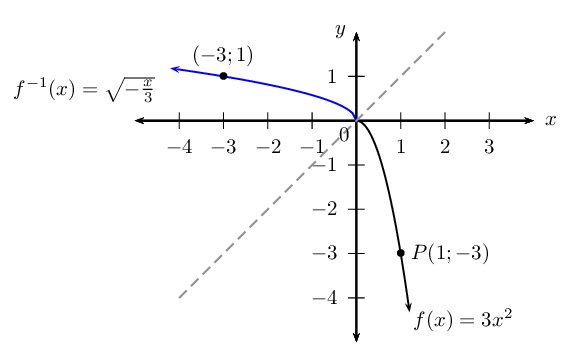Determine the inverse of $$h(x) = \frac{11}{5}x^{2}$$.

\begin{align*} \text{Let } y & = \frac{11}{5}x^{2} \\ \text{Interchange } x \text{ and } y: \quad x & = \frac{11}{5}y^{2} \\ \frac{5}{11} & = y^{2} \\ \therefore y & = ±\sqrt{\frac{5}{11}x} \qquad (x \geq 0) \end{align*}

Sketch both graphs on the same system of axes.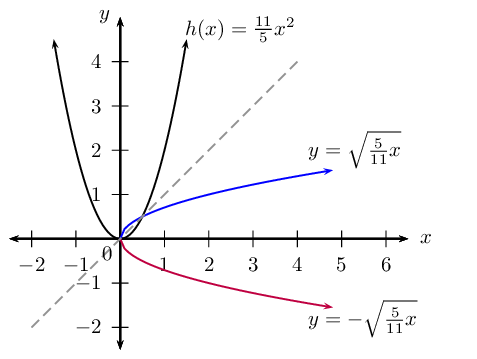Restrict the domain of $$h$$ so that the inverse is a function.

Option $$\text{1}$$: Restrict the domain of $$h$$ to $$x \ge 0$$ so that the inverse will also be a function $$\left( h^{-1} \right)$$. The restriction $$x \ge 0$$ on the domain of $$h$$ will restrict the range of $$h^{-1}$$ such that $$y \ge 0$$.

\begin{align*} h: \qquad & \text{domain } x \ge 0 \quad \text{range } y \ge 0 \\ h^{-1}: \qquad & \text{domain } x \ge 0 \quad \text{range } y \ge 0 \end{align*}or

Option $$\text{2}$$: Restrict the domain of $$h$$ to $$x \le 0$$ so that the inverse will also be a function $$\left( h^{-1} \right)$$. The restriction $$x \le 0$$ on the domain of $$h$$ will restrict the range of $$h^{-1}$$ such that $$y \le 0$$.

\begin{align*} h: \qquad & \text{domain } x \le 0 \quad \text{range } y \ge 0 \\ h^{-1}: \qquad & \text{domain } x \ge 0 \quad \text{range } y \le 0 \end{align*}The diagram shows the graph of $$g(x) = mx + c$$ and $$f^{-1}(x) = a \sqrt{x}, \enspace (x \geq 0)$$. Both graphs pass through the point $$P(4;-1)$$.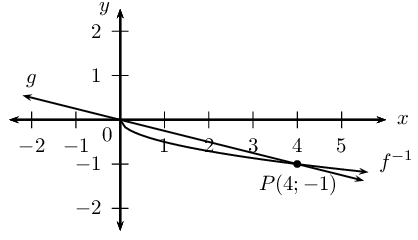Determine the values of $$a$$, $$c$$ and $$m$$.

From the graph we see that the straight line passes through the origin, therefore $$c=0$$.

\begin{align*} g(x) & = mx \\ \text{Substitute } P(4;-1): \enspace -1 & = 4m \\ \therefore m & = - \frac{1}{4} \\ \therefore g(x) & = - \frac{1}{4} x \\ & \\ f^{-1}(x)& = a \sqrt{x} \\ \text{Substitute } P(4;-1): \enspace -1 & = a \sqrt{4} \\ -1 & = 2a \\ \therefore a & = - \frac{1}{2} \\ \therefore f^{-1}(x) & = - \frac{1}{2} \sqrt{x} \end{align*}

Give the domain and range of $$f^{-1}$$ and $$g$$.

\begin{align*} g: \qquad & \text{domain: } x \in \mathbb{R} \quad \text{range: } y \in \mathbb{R} \\ f^{-1}: \qquad & \text{domain: } x \ge 0 \quad \text{range: } y \le 0 \end{align*}

For which values of $$x$$ is $$g(x) < f(x)$$?

$$x > 4$$

Determine $$f$$.

\begin{align*} \text{Let } y & = - \frac{1}{2} \sqrt{x} \\ \text{Interchange } x \text{ and } y: \quad x & = - \frac{1}{2} \sqrt{y} \qquad (y \geq 0) \\ -2x & = \sqrt{y} \\ \therefore y & = 4x^{2} \qquad (y \geq 0) \end{align*}

Determine the coordinates of the point(s) of intersection of $$g$$ and $$f$$ intersect.

To determine the coordinates of the point(s) of intersection, we equate $$g$$ and $$f$$:

\begin{align*} - \frac{1}{4} x & = 4x^{2} \\ 0 & = 4x^{2} + \frac{1}{4} x \\ 0 & = x \left(4x + \frac{1}{4} \right) \\ \therefore x = 0 & \text{ or } 4x + \frac{1}{4} = 0 \\ \text{If } x = 0: \enspace y &= 0 \\ \text{If } 4x + \frac{1}{4} &= 0: \\ 4x &= - \frac{1}{4} \\ x &= - \frac{1}{16} \\ \text{If } x = - \frac{1}{16}: \enspace y &= - \frac{1}{4} \left( - \frac{1}{16} \right) \\ &= \frac{1}{64} \end{align*}

Therefore, the two graphs intersect at $$\left( 0;0 \right)$$ and $$\left( - \frac{1}{16}; \frac{1}{64} \right)$$.

## Worked example 9: Inverses - average gradient

Given: $$h(x) = 2x^{2}, \quad x \ge 0$$

1. Determine the inverse, $$h^{-1}$$.
2. Find the point where $$h$$ and $$h^{-1}$$ intersect.
3. Sketch $$h$$ and $$h^{-1}$$ on the same set of axes.
4. Use the sketch to determine if $$h$$ and $$h^{-1}$$ are increasing or decreasing functions.
5. Calculate the average gradient of $$h$$ between the two points of intersection.

### Determine the inverse of the function

\begin{align*} \text{Let } y & = 2x^{2} \qquad (x \ge 0) \\ \text{Interchange } x \text{ and } y: \quad x & = 2y^{2} \qquad (y \ge 0) \\ \frac{x}{2} & = y^{2} \\ y & = \sqrt{\frac{x}{2}} \qquad (x \ge 0, y \geq 0) \\ & \\ \therefore h^{-1}(x) & = \sqrt{\frac{x}{2}} \qquad (x \geq 0) \end{align*}

### Determine the point of intersection

\begin{align*} 2x^{2} & = \sqrt{\frac{x}{2}} \\ \left( 2x^{2} \right)^{2} & = \left( \sqrt{\frac{x}{2}} \right)^{2} \\ 4x^{4} & = \frac{x}{2} \\ 8x^{4} & = x \\ 8x^{4} - x & = 0 \\ x(8x^{3} - 1) & = 0 \\ \therefore x = 0 &\text{ or } \enspace 8x^{3} - 1 = 0 \\ \text{If } x=0, \quad y & = 0 \\ \text{If } 8x^{3} - 1 & = 0 \\ 8x^{3} & = 1 \\ x^{3} & = \frac{1}{8} \\ \therefore x & = \frac{1}{2} \\ \text{If } x= \frac{1}{2}, \quad y & = \frac{1}{2} \end{align*}

Therefore, this gives the points A$$(0;0)$$ and $$B\left( \frac{1}{2} ; \frac{1}{2}\right)$$.

### Sketch both graphs on the same system of axes### Examine the graphs

From the graphs, we see that both $$h$$ and $$h^{-1}$$ pass the vertical line test and therefore are functions.

\begin{align*} h: \quad & \text{as } x \text{ increases, } y \text{ also increases, } \text{ therefore } h \text{ is an increasing function. } \\ h^{-1}: \quad & \text{as } x \text{ increases, } y \text{ also increases, } \text{ therefore } h^{-1} \text{ is an increasing function. } \end{align*}

Calculate the average gradient of $$h$$ between the points A$$(0;0)$$ and $$B\left( \frac{1}{2} ; \frac{1}{2}\right)$$.

\begin{align*} \text{Average gradient: } &= \frac{y_{B} - y_{A}}{x_{B} - x_{A}} \\ &= \frac{ \frac{1}{2} - 0 }{\frac{1}{2} - 0 } \\ &= 1 \end{align*}

Note: this is also the average gradient of $$h^{-1}$$ between the points $$A$$ and $$B$$.

## Inverses - average gradient, increasing and decreasing functions

Textbook Exercise 2.5

Sketch the graph of $$y={x}^{2}$$ and label a point other than the origin on the graph.Find the equation of the inverse of $$y = x^{2}$$.

\begin{align*} y & = x^{2} \\ \text{Inverse: } x & = y^{2} \\ \therefore y & = \pm \sqrt{x} \qquad (x \ge 0) \end{align*}

Sketch the graph of the inverse on the same system of axes.No. For certain values of $$x$$, the inverse cuts a vertical line in two places. Therefore, it is not function.

$$P(2;4)$$ is a point on $$y = x^{2}$$. Determine the coordinates of $$Q$$, the point on the graph of the inverse which is symmetrical to $$P$$ about the line $$y = x$$.

$$Q(4;2)$$

1. the origin and $$P$$;
2. the origin and $$Q$$.

1. \begin{align*} \text{Average gradient: } &= \frac{y_{P} - y_{O}}{x_{P} - x_{O}} \\ &= \frac{ 4 - 0 }{2- 0 } \\ &= 2 \end{align*}
2. \begin{align*} \text{Average gradient: } &= \frac{y_{Q} - y_{O}}{x_{Q} - x_{O}} \\ &= \frac{ 2 - 0 }{4- 0 } \\ &= \frac{1}{2} \end{align*}

$$\text{Average gradient}_{ OP} = 2$$ and $$\text{average gradient}_{ OQ} = \frac{1}{2}$$.

Both gradients are positive, and they are also reciprocals of each other.

Given the function $$f^{-1}(x) = kx^{2}$$, $$x \ge 0$$, which passes through the point $$P \left( \frac{1}{2}; -1 \right)$$.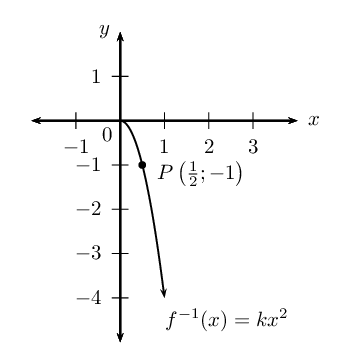Find the value of $$k$$.
\begin{align*} f^{-1}(x) & = kx^{2} \\ \text{Substitute } \left( \frac{1}{2}; -1 \right) \quad -1 & = k \left( \frac{1}{2} \right)^{2} \\ -1 & = k \left( \frac{1}{4} \right) \\ -4 & = k \\ \therefore f^{-1}(x) & = -4x^{2} \end{align*}

State the domain and range of $$f^{-1}$$.

\begin{align*} \text{Domain: } & \{x: x \ge 0, x \in \mathbb{R} \} \\ \text{Range: } & \{y: y \le 0, y \in \mathbb{R} \} \end{align*}

Find the equation of $$f$$.

\begin{align*} f^{-1}: \quad y & = -4x^{2} \quad (x \ge 0) \\ f: \quad x & = -4y^{2} \quad (y \ge 0) \\ -\frac{1}{4}x & = y^{2} \\ \therefore y & = \sqrt{-\frac{1}{4}x} \quad (x \le 0) \end{align*}

State the domain and range of $$f$$.

\begin{align*} \text{Domain: } & \{x: x \le 0, x \in \mathbb{R} \} \\ \text{Range: } & \{y: y \ge 0, y \in \mathbb{R} \} \end{align*}

Sketch the graphs of $$f$$ and $$f^{-1}$$ on the same system of axes.Is $$f$$ an increasing or decreasing function?

Decreasing function: as the value of $$x$$ increases, the function value decreases.

Given: $$g(x) = \frac{5}{2}x^{2}, \enspace x \ge 0$$.

Find $$g^{-1}(x)$$.

\begin{align*} \text{Let } y & = \frac{5}{2}x^{2} \qquad (x \ge 0) \\ \text{Interchange } x \text{ and } y: \quad x & = \frac{5}{2}y^{2} \qquad (y \ge 0) \\ \frac{2}{5}x & = y^{2} \\ y & = \sqrt{\frac{2}{5}x} \qquad (x \ge 0, y \geq 0) \\ & \\ \therefore g^{-1}(x) & = \sqrt{\frac{2}{5}x} \qquad (x \geq 0) \end{align*}

Calculate the point(s) where $$g$$ and $$g^{-1}$$ intersect.

\begin{align*} \frac{5}{2}x^{2} & = \sqrt{\frac{2}{5}x} \\ \left( \frac{5}{2}x^{2} \right)^{2} & = \left( \sqrt{\frac{2}{5}x} \right)^{2} \\ \frac{25}{4}x^{4} & = \frac{2}{5}x \\ \frac{25}{4}x^{4} - \frac{2}{5}x & = 0 \\ 125x^{4} - 8x & = 0 \\ x(125x^{3} - 8) & = 0 \\ x(5x - 2)(25x^{2} +10x + 4) & = 0 \\ \therefore x = 0 \enspace \text{ or } \enspace 5x - 2 = 0 \enspace &\text{ or } \enspace 25x^{2} +10x + 4 = 0 \\ \text{If } x=0, \quad y & = 0 \\ \text{If } x = \frac{2}{5}, \quad y & = \frac{5}{2} \left( \frac{2}{5} \right) ^{2} \\ \therefore y & = \frac{2}{5} \\ \text{If } 25x^{2} +10x + 4 &=0 \\ \text{Use quadratic formula: } \quad x &= \frac{-10 \pm \sqrt{100 - 4(25)(4)}}{2(25)}\\ &= \frac{-10 \pm \sqrt{-300}}{50} \\ \therefore & \text{no real solution} \end{align*}

Therefore, the points of intersection are $$(0;0)$$ and $$\left( \frac{2}{5};\frac{2}{5} \right)$$.

Sketch $$g$$ and $$g^{-1}$$ on the same set of axes.Use the sketch to determine if $$g$$ and $$g^{-1}$$ are increasing or decreasing functions.

\begin{align*} g: \enspace & \text{as } x \text{ increases, } y \text{ also increases, } \therefore g \text{ is an increasing function. } \\ g^{-1}: \enspace & \text{as } x \text{ increases, } y \text{ also increases, } \therefore g^{-1} \text{ is an increasing function. } \end{align*}

Calculate the average gradient of $$g^{-1}$$ between the two points of intersection.

\begin{align*} \text{Average gradient: } &= \frac{y_{2} - y_{1}}{x_{2} - x_{1}} \\ &= \frac{ \frac{2}{5} - 0 }{\frac{2}{5} - 0 } \\ &= 1 \end{align*}